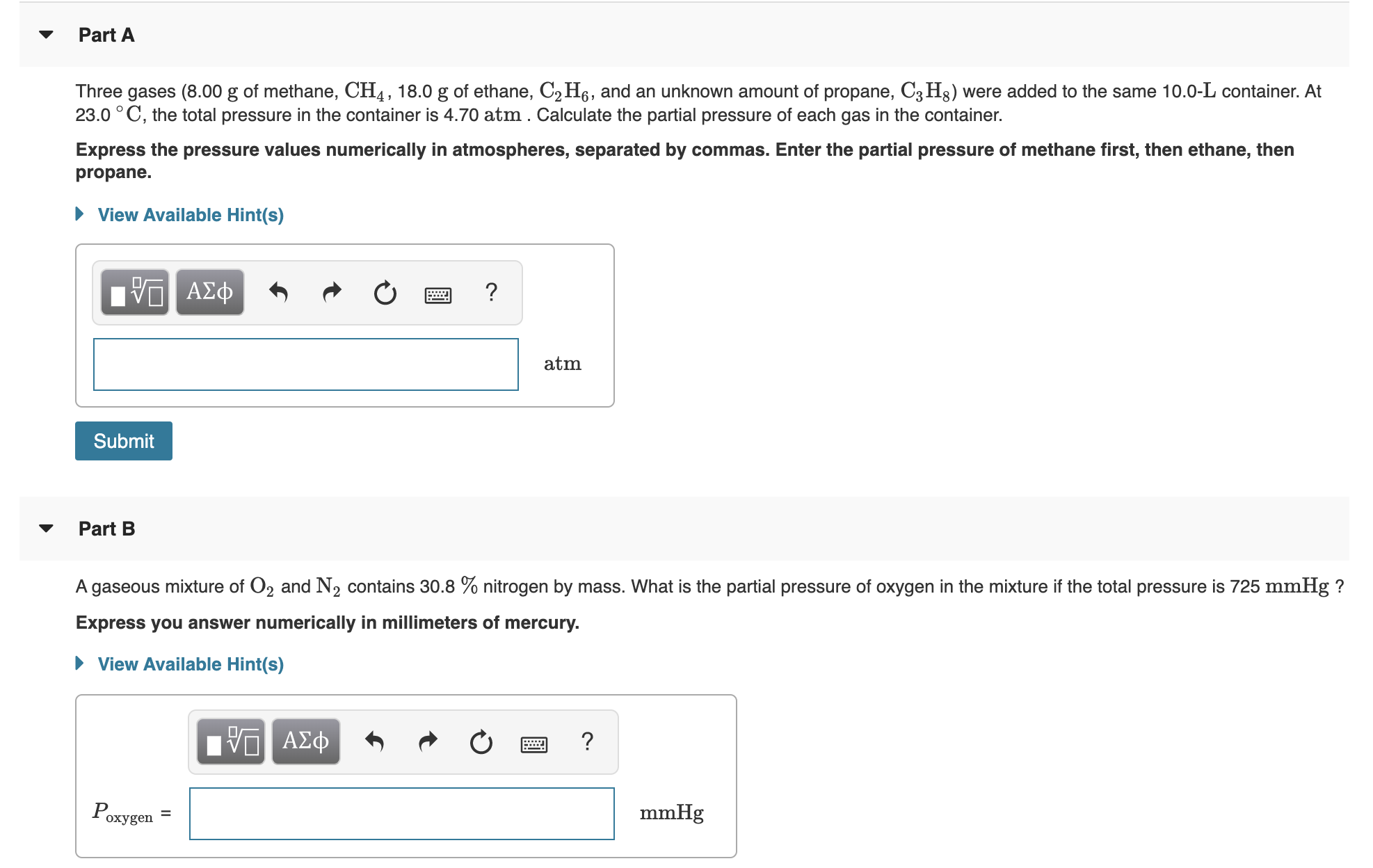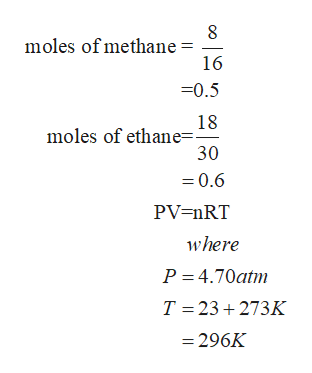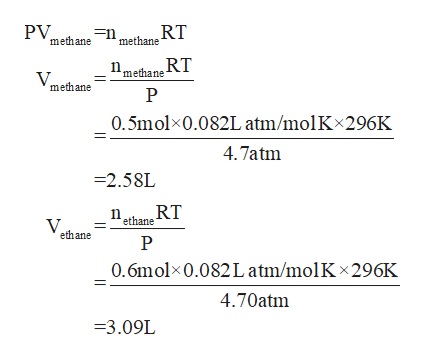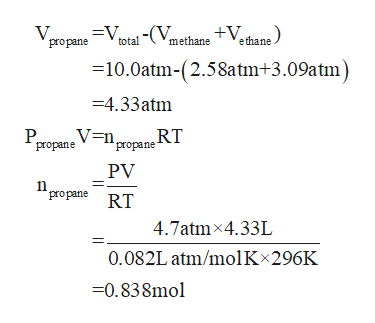# Part AThree gases (8.00 g of methane, CH4, 18.0 g of ethane, C2 H6, and an unknown amount of propane, C3H8) were added to the same 10.0-L container. At23.00 C, the total pressure in the container is 4.70 atm . Calculate the partial pressure of each gas in the container.OExpress the pressure values numerically in atmospheres, separated by commas. Enter the partial pressure of methane first, then ethane, thenpropane.View Available Hint(s)VADatmSubmitPart BA gaseous mixture of O2 and N2 contains 30.8 % nitrogen by mass. What is the partial pressure of oxygen in the mixture if the total pressure is 725 mmHg?Express you answer numerically in millimeters of mercuryView Available Hint(s)ADmmHgOxygen

Question
118 viewshelp_outlineImage TranscriptionclosePart A Three gases (8.00 g of methane, CH4, 18.0 g of ethane, C2 H6, and an unknown amount of propane, C3H8) were added to the same 10.0-L container. At 23.00 C, the total pressure in the container is 4.70 atm . Calculate the partial pressure of each gas in the container. O Express the pressure values numerically in atmospheres, separated by commas. Enter the partial pressure of methane first, then ethane, then propane. View Available Hint(s) VAD atm Submit Part B A gaseous mixture of O2 and N2 contains 30.8 % nitrogen by mass. What is the partial pressure of oxygen in the mixture if the total pressure is 725 mmHg? Express you answer numerically in millimeters of mercury View Available Hint(s) AD mmHg Oxygen fullscreen
check_circle

Step 1

To calculate the unknown amount of propanehelp_outlineImage Transcriptionclosemoles of methane 16 0.5 18 moles of ethane=. 30 0.6 PV=nRT where P 4.70atm T 23 273K = 296K fullscreen
Step 2

Calculating the individual volumes occupied by these gaseshelp_outlineImage TranscriptionclosePV methane RT =n methane methane RT P V methane 0.5molx0.082L atm/molKx296K 4.7atm -2.58L RT ethane ethane 0.6molx0.082L atm/molK x 296K 4.70atm 3.09L fullscreen
Step 3

The volume occupied by propane ...help_outlineImage TranscriptioncloseV propane Vptal -(VmethaneV¢hane) total =10.0atm-(2.58atm+3.09atm =4.33atm P Vn 'propane RT propane PV propane RT 4.7atm 4.33L 0.082L atm/molKx296K 0.838mol fullscreen

### Want to see the full answer?

See Solution

#### Want to see this answer and more?

Solutions are written by subject experts who are available 24/7. Questions are typically answered within 1 hour.*

See Solution
*Response times may vary by subject and question.
Tagged in

### Chemistry